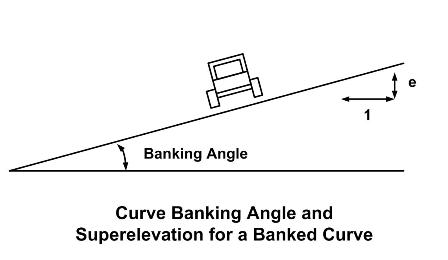* Log In to use the Calculate function * Become a Member!

Calculate Curve Banking Angle and Superelevation (Pavement Cross Slope):

Calculation:
Designer/Checker:

Input:Design Speed (MPH - mph or kph)
Radius of curve (R - ft or m)

Output:

##### Login to enable the Formulas!

Banking Angle =
Superelevation, e =

For MPH in mph:
If MPH < 30, f = 0.16
If 50 >MPH >= 30, f = 0.16-0.01((MPH-30)/10)
If MPH >= 50, f = 0.14-0.02((MPH-50)/10)
*AASHTO recommends maximum e = 0.08

 Start Using The Calculations >>> Become A Member Now!